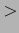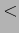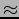\$B \$BI>2A7k2L(B \$B>e\$X(B: \$B?M2A(B \$BLa\$k(B: \$B?M2A(B   \$BL\

## \$BH=CG4p=`(B

\$B?M2A\$r9T\$&!%I>2A4p=`\$HI>2ANc\$r0J2<\$K<((B \$B\$9!%>0!\$L\$CN8l\$O%m!<%^;zJQ49\$7\$FI>2A\$9\$k!%(B

\$BI>2A(B1() \$BDs0F

 \$BNc(B1 \$BF~NOJ8(B \$B;d(B \$B\$O(B \$BH`=w(B \$B\$K(B \$B7k:'(B \$B\$r(B \$B?=\$79~\$s(B \$B\$@(B \$B!#(B \$B@52rJ8(B I proposed to her . \$B%Y!<%9%i%\$%s(B I He asked her for her hand . \$BDs0F I made a proposal of marriage to her . \$BNc(B2 \$BF~NOJ8(B \$BH`(B \$B\$O(B \$B%9%i%\$%I(B \$B\$r(B \$B%9%/%j!<%s(B \$B\$K(B \$B1G\$7(B \$B\$?(B \$B!#(B \$B@52rJ8(B He projected the slides on the screen . \$B%Y!<%9%i%\$%s(B He looked at herself in the screen . \$BDs0F He projected a slide The picture on the TV screen .

\$BI>2A(B2() \$BDs0F
 \$BNc(B1 \$BF~NOJ8(B \$B;E;v(B \$B\$O(B \$B;3>l(B \$B\$K(B \$BF~\$C(B \$B\$?(B \$B!#(B \$B@52rJ8(B Work has reached the critical point . \$B%Y!<%9%i%\$%s(B The work is appear to have entered the final stage . \$BDs0F work went into the labor-management . \$BNc(B2 \$BF~NOJ8(B \$BBf=j(B \$B\$N(B \$B6u5\$(B \$B\$,(B \$B1x\$l(B \$B\$F(B \$B\$\$\$k(B \$B!#(B \$B@52rJ8(B The air in the kitchen is foul . \$B%Y!<%9%i%\$%s(B The air is polluted the kitchen . \$BDs0F The kitchen is the dirt off the air .

\$BI>2A(B3() \$B\$I\$A\$i\$b;w\$?\$h\$&\$JJ8\$G\$"\$k!\$\$^\$?\$O\$I\$A\$i\$bF~NOJ8\$GEA\$(\$?\$\$>pJs\$,M}2r\$G\$-\$J\$\$(B

 \$BNc(B1 \$BF~NOJ8(B \$BK-:n(B \$B\$K(B \$B\$J\$j(B \$B\$=\$&(B \$B\$@(B \$B!#(B \$B@52rJ8(B The harvest looks promising . \$B%Y!<%9%i%\$%s(B Hopes looks like . \$BDs0F It looks like rejoicing . \$BNc(B2 \$BF~NOJ8(B \$B\$=\$l(B \$B\$O(B \$BH`(B \$B\$N(B \$BD&9o(B \$B\$7(B \$B\$?(B \$B\$b\$N(B \$B\$@(B \$B!#(B \$B@52rJ8(B It is a production of his chisel . \$B%Y!<%9%i%\$%s(B It will be a is engraved . \$BDs0F It is engraved .

\$BI>2A(B4(=) \$BDs0F
 \$BNc(B1 \$BF~NOJ8(B \$BH`(B \$B\$O(B \$B8N6?(B \$B\$r(B \$BNx\$7(B \$B\$,\$C(B \$B\$F(B \$B\$\$\$k(B \$B!#(B \$B@52rJ8(B He is sick for home . \$B%Y!<%9%i%\$%s(B He is homesick . \$BDs0F He is homesick . \$BNc(B2 \$BF~NOJ8(B \$B45 \$B@52rJ8(B The patient was operated on for appendicitis . \$B%Y!<%9%i%\$%s(B The patient had an operation for appendicitis . \$BDs0F The patient had an operation for appendicitis .\$B \$BI>2A7k2L(B \$B>e\$X(B: \$B?M2A(B \$BLa\$k(B: \$B?M2A(B   \$BL\
\$BJ?@.(B22\$BG/(B2\$B7n(B11\$BF|(B